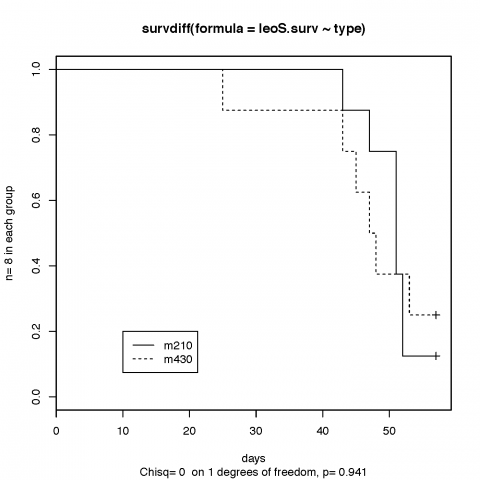Want to share your content on R-bloggers? click here if you have a blog, or here if you don't.

Leonid asked me to do a quick survival analysis of two different types of mouse (m430 and m210) with surgically implanted tumours (or something like that). The data was in the wrong format but after transforming it looked like this:In my opinion there are far too few samples to detect any significant difference between the mouse types in anything but the most extreme case. Nevertheless it is useful for him to go through the motions and publish what he has….or rather what I do for him.

I used the survival package for R . Here is the script and results.

##attach column names to the search path
> attach(leoS)

## make a survival object
> leoS.surv= Surv(time,delta)

##fit the data separating by mouse type
> my.fit= survfit(leoS.surv~type)

## use the G-rho tests for difference between the curves
> sdf= survdiff(leoS.surv~type)

## survdiff( ) is described here: Harrington, D. P. and Fleming, T. R. (1982). A class of rank test procedures for censored survival data.

>sdf
Call:
survdiff(formula = leoS.surv ~ type)

N Observed Expected (O-E)^2/E (O-E)^2/V
type=m210 8        7     7.12   0.00208   0.00543
type=m430 8        6     5.88   0.00252   0.00543

Chisq= 0  on 1 degrees of freedom, p= 0.941

## and now plot it all together

> plot(my.fit, lty=1:2, mark.time=T)
> legend(10, .2, c(“m210”, “m430”), lty=1:2)
> title(main=’survdiff(formula = leoS.surv ~ type)’, sub=’Chisq= 0  on 1 degrees of freedom, p= 0.941′, ylab=”n= 8 in each group”, xlab=”days”)

Which gives….which I think is good enough for such a small experiment. I saved the data, images and RData in Desktop/Projects/Leonid/mouse_survival.